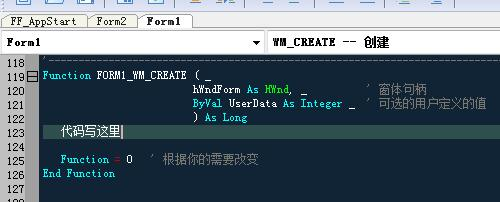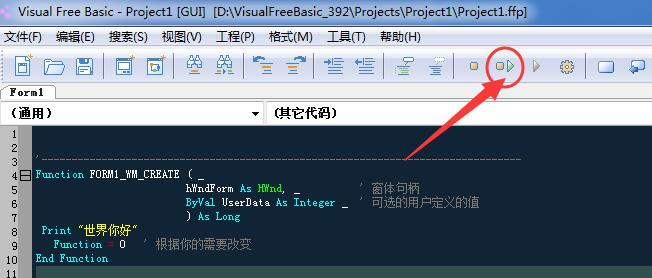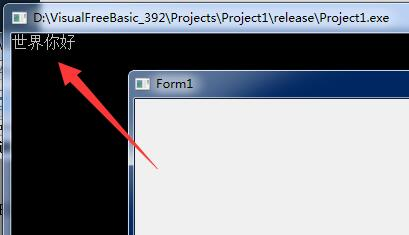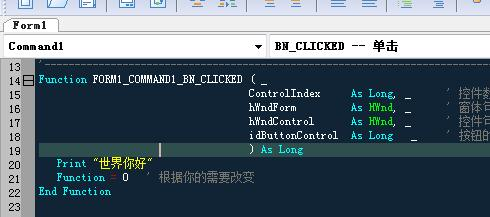﻿ FreeBASIC语言入门（VFB教程2-1）-编程-勇芳软件开发小组FreeBASIC语言入门（VFB教程2-1）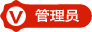2018-2-16 9640

Dim

Print

For...Next

If...Then

Do...Loop

Print "你好，世界！"

Dim text As String
text = "你好，世界！"
Print text

Dim a As String, b As String, text As String
a = "你好"
b = "世界"
text = a + " " + b + "!"
Print text

Dim a As Integer, b As Integer, c As Integer

a = 5
b = 7
c = a + b

Print "a = "; a
Print "a = "; b
Print "a + b = "; c

Dim total As Integer
Dim number As Integer
total = 0
For number = 1 To 100
total = total + number
Next
Print "The sum of number from 1 to 100 is "; total

Dim number As Integer
number=1
Print "Your number is ";
If number < 0 Then
Print "negative"
ElseIf number > 0 Then
Print "positive"
Else
Print "zero"
End If

Dim total As Single, count As Single, number As Single
Dim text As String

Print "This program will calculate the sum and average for a"
Print "list of numbers.  Enter an empty value to end."
Print

Do
Input "Enter a number : ", text
If text = "" Then
Exit Do
End If

count = count + 1
total = total + Val(text)

Loop

Print
Print "You entered "; count; " numbers"
Print "The sum is "; total
If count <> 0 Then
Print "The average is "; total / count
End If